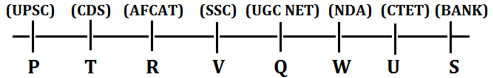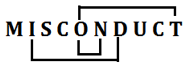Latest Banking jobs   »

# Reasoning Ability Quiz For SIDBI GRADE A 2023- 7th January

Directions (1-5): Study the following information carefully and answer the question given below.
Eight persons are sitting in a straight line and all are facing north direction. Each of them prepares for different competitive exam. S prepares for Bank and sits third to the right of Q. R sits immediate left of V who prepares for SSC. The one who prepares for AFCAT sits second to the left of Q. The one who prepares for UPSC sits third to the left of V. W prepares for NDA and he is an immediate neighbour of U who does not prepare for AFCAT. V is not an immediate neighbour of S. Persons who prepare for CDS and UPSC sit together. T sits third to the left of the one who prepares for UGC NET. W is not an immediate neighbour of S. P does not prepare for CTET.

Q1. Who among the following person prepare for CTET exam?
(a) T
(b) R
(c) W
(d) U
(e) None of these

Q2. Who among the following person sits third to the right V?
(a) The one who prepares for AFCAT
(b) The one who prepares for CTET
(c) S
(d) P
(e) None of these

Q3. What is the position of Q with respect to T?
(a) Third to the right
(b) Immediate Left
(c) Second to the right
(d) Immediate Right
(e) None of these

Q4. Which of the following statement is not true about U?
(a) U prepares for CTET
(b) U sits immediate right of S
(c) U does not prepare for UPSC
(d) Only two persons sit between U and the one who prepares for SSC
(e) Both (B) and (D)

Q5. Who among the following sits immediate right of the one who prepares for UGC NET?
(a) V
(b) U
(c) W
(d) P
(e) None of these

Directions (6-10): In these questions, relationship between different elements is shown in the statements. The statements are followed by conclusions. Study the conclusions based on the given statements and select the appropriate answer:

Q6. Statements: G>H≤A=W≥U>O>D>C
Conclusion I: H<W
II: A>D
(a) If only conclusion I follows.
(b) If only conclusion II follows.
(c) If either conclusion I or II follows
(d) If neither conclusion I nor II follows.
(e) If both conclusions I and II follow.

Q7. Statements: J>W=X>I<P=D>L<F≤R
Conclusion I: X=L
II: F>P
(a) If only conclusion I follows.
(b) If only conclusion II follows.
(c) If either conclusion I or II follows
(d) If neither conclusion I nor II follows.
(e) If both conclusions I and II follow.

Q8. Statements: W≤E≤U>C<J≤D<L=S≤I
Conclusion I: C<S
II: U<D
(a) If only conclusion I follows.
(b) If only conclusion II follows.
(c) If either conclusion I or II follows
(d) If neither conclusion I nor II follows.
(e) If both conclusions I and II follow.

Q9. Statements: X≤W=S>D=M>K>O≤P≤L
Conclusion I: S≤O
II: S>O
(a) If only conclusion I follows.
(b) If only conclusion II follows.
(c) If either conclusion I or II follows
(d) If neither conclusion I nor II follows.
(e) If both conclusions I and II follow.

Q10. Statements: Q≤H=X>M≤O<P<L=D>H
Conclusion I: D>M
II: X>L
(a) If only conclusion I follows.
(b) If only conclusion II follows.
(c) If either conclusion I or II follows
(d) If neither conclusion I nor II follows.
(e) If both conclusions I and II follow.

Q11. The position of how many alphabets will remain unchanged if each of the alphabets in the word ‘NEWSLETTER’ is arranged in alphabetical order from left to right?
(a) Four
(b) One
(c) Three
(d) Two
(e) None

Q12. If the expressions ‘M>N ≤ O < P’, ‘N >Y’ and ‘P <Q’ are true, which of the following conclusions will be false?
(a) N < Q
(b) M> Q
(c) Y < M
(d) O > Y
(e) None of these

Q13. In a class, all students are seating on a bench and facing north, Meena is 14th from left and Sneha is 10th from right end. Then how many children are seating between Meena and Sneha?
(a) 4
(b) 7
(c) 5
(e) 10

Q14. How many such pairs of letters are there in the word ‘MISCONDUCT’ each of which has as many letters between them in the word as in the English alphabet?
(a) One
(b) None
(c) Two
(d) Three
(e) More than three

Q15. In a number 86143259 if all digits are arranged in ascending order from left to right within the number, then how many digits will remain on the same position?
(a) One
(b) None
(c) three
(d) Two
(e) Four

Solutions

Solution (1-5)S1. Ans. (d)
S2. Ans. (b)
S3. Ans. (a)
S4. Ans. (b)
S5. Ans. (c)

S6. Ans. (b)
Sol. I: H<W (false)
II: A>D(True)

S7. Ans. (d)
Sol. I: X=L (False)
II: F>P(False)

S8. Ans. (a)
Sol. I: C<S(True)
II: U<D(False)

S9. Ans. (b)
Sol. I: S≤O (False)
II: S>O(True)

S10. Ans. (a)
Sol. I: D>M(True)
II: X>L (False)

S11. Ans. (d)
Obtained Word- EEELNRSTTW

S12. Ans. (b)

S13. Ans. (d)

S14. Ans. (d)
Sol.S15. Ans. (d)
Sol. Original number- 86143259
New number-12345689## FAQs

### Q1. Who among the following person prepare for CTET exam? (a) T (b) R (c) W (d) U (e) None of these

S1. Ans. (d)

#### Congratulations!